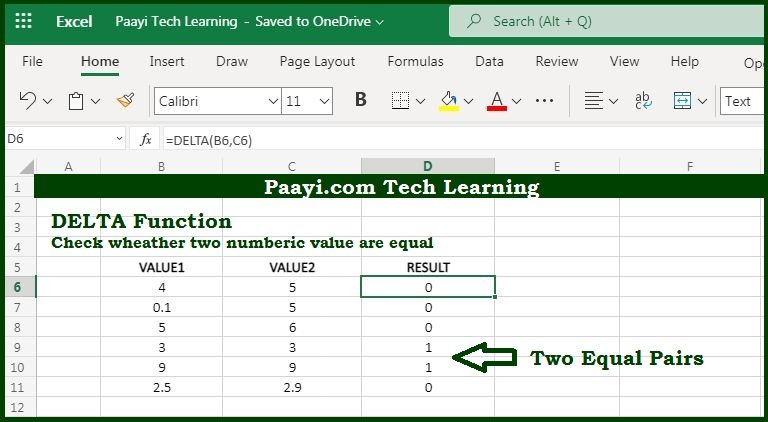# Learn How to use Microsoft Excel DELTA Function

Written by | 0 Comments | 650 Views

In this article, you will learn how to use the Microsoft Excel DELTA function and its prime function in Microsoft Excel. You will also get to know the Microsoft Excel DELTA function return value and syntax with the help of some examples.

Microsoft Excel DELTA Function

The prime function of the Microsoft Excel DELTA function is to test two values are equal. That means with the help of the DELTA function you can able to test if the two numeric values are equal or not. When the DELTA function finds the two values equal, it will return 1, otherwise 0.

Return Value of DELTA Function

The return value will be number one or zero.

Syntax of DELTA Function

=DELTA(number1, [number2])

Where the arguments:

• number1: It is the first number.
• number2: It is the second number (optional).

## How to Use Microsoft Excel DELTA Function?As we know that DELTA function used to check the equality of the two numbers, in case the values are equal the DELTA function return 1 otherwise returns 0. That means you can use the DELTA function to easily count the pair of equal numbers.

If you left the number2 blank, the function assumes it as zero. If any one of the values is text the function returns the error as #VALUE.